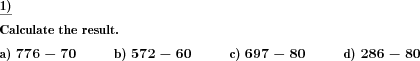Custom math worksheets at your fingertips# Details for problem "Subtract mupltiples of powers of ten"

Quickname: 6761

Elementary School, Primary School, Middle School.

## Summary

Multiples of powers of ten subtracted from natural numbers.

## Example## Description

In this subtraction problem, the minuend is a natural positive number with a defined number of places. The subtrahend is a multiple of a power of ten. That means that the subtrahend's first digit will be in the range 1-9, while the other ones will be zero. The subtrahend will always have fewer places than the minuend.

Borrows will never occur.

The number of places for both numbers can be specified. It can be specified that the first number will only have zeroes in the range of places the second number covers.

The number of problems can be selected.

Download free printable worksheets for this math problem here. The worksheet contains the problems only, the solution sheet includes the answers. Just click on the respective link.

•Worksheet 1Solution sheet with answers
•Worksheet 2Solution sheet with answers
•Worksheet 3Solution sheet with answers

If you can not see the solution sheets for download, they may be filtered out by an ad blocker that you may have installed. If this is the case, please allow ads for this page and reload the page. The solution sheets will then reappear.

• Do these sample worksheets do not really fit?
• Do you need more math worksheets, with a different level of difficulty?
• Would you like to combine different problems on a worksheet and adjust them to your needs?
• As a teacher, you can put together your own worksheets using the automatically generated math problems provided.
With a free initial credit, you can start creating your own math worksheets in a few minutes.

You can try it for free! Register here, to create custom worksheets now!

## Customization options for this problem

Parameter
Possible values
Number of problems
1, 2, 3, 4, 5, 6, 7, 8, 9, 10
Minuend places
1, 2, 3, 4, 5, 6
Subtrahend places
1, 2, 3, 4, 5, 6
Rightmost places minuend>0
Yes, No